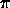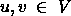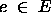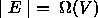CSE 5311 Section 501 Fall 1998
Homework 6
Due: April 15, 1998, in class

1. Run Dijkstra's algorithm on the directed graph shown in Figure 25.2 in the textbook, first using vertex s as the source and then using vertex y as the source. Show the d andvalues and the vertices in set S after each iteration of the while loop (this is Exercise 25.2-1 in the textbook).
2. Run the Bellman-Ford algorithm on the directed graph shown in Figure 25.7 in the textbook using vertex y as the source. Relax edges in lexicographic order (see section 13-2 for a description of this concept) in each pass, and show the d andvalues after each pass. Now, change the weight of edge (y,v) to 4 and run the algorithm again, using z as the source (this is Exercise 25.3-1 in the textbook).
3. Given a weighted, directed graph G = (V, E) with no negative-weight cycles, let m be the maximum over all pairs of verticesof the minimum number of edges in a shortest path from u to v. In this case the shortest path is by weight, not by the number of edges. Suggest a change to the Bellman-ford algorithm that allows it to terminate in m+1 passes (this is Exercise 25.3-3 in the textbook).
4. How can the output of the Floyd-Warshall algorithm be used to detect the presence of a negative-weight cycle? This is Exercise 26.2-5 in the textbook.
5. Let G = (V,E) be a flow network with source s, sink t, and suppose each edgehas capacity c(e) = 1. Assume also, for convenience, that.
Suppose a maximum flow for G has been computed, and a new edge with capacity 1 is added to E. Describe how the maximum flow can be efficiently updated. It is not the value of the flow that must be updated, but the flow itself. Analyze the run time of your algorithm.
6. Consider a parallel version of the Bellman-Ford algorithm (serial pseudocode shown below) where the for loops beginning at lines 3 and 5 are executed in parallel (i.e., constant time).

```Initialize-Single-Source(G,s)
for  each vertex v in V[G]
d[v] = infinity
pi[v] = nil
d[s] = 0
Relax(u,v,w)
if d[v] > d[u] + w(u,v)
then d[v] = d[u] + w(u,v)
pi[v] = u

Bellman-Ford(G,w,s)
1.    Initialize-Single-Source(G,s)
2.    for i = 1 to |V[G]| - 1
3.       for each edge (u,v) in E[G]
4.          Relax(u,v,w)
5.       for each edge (u,v) in E[G]
6.          if d[v] > d[u] + w(u,v)
7.          then return {\sc false}
8.    return true```

What is the running time, the speedup, and the efficiency of the parallel algorithm?

Diane J. Cook
Mon Feb 2 21:56:55 CST 1998